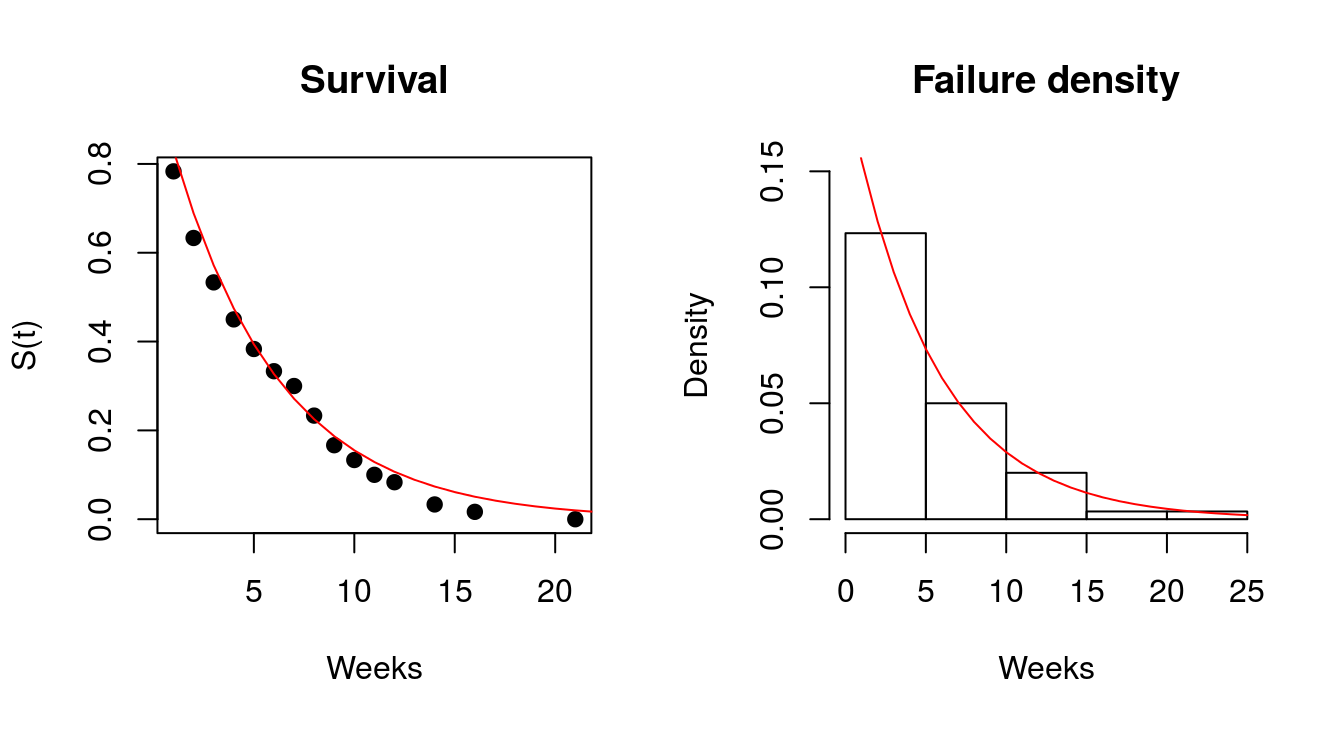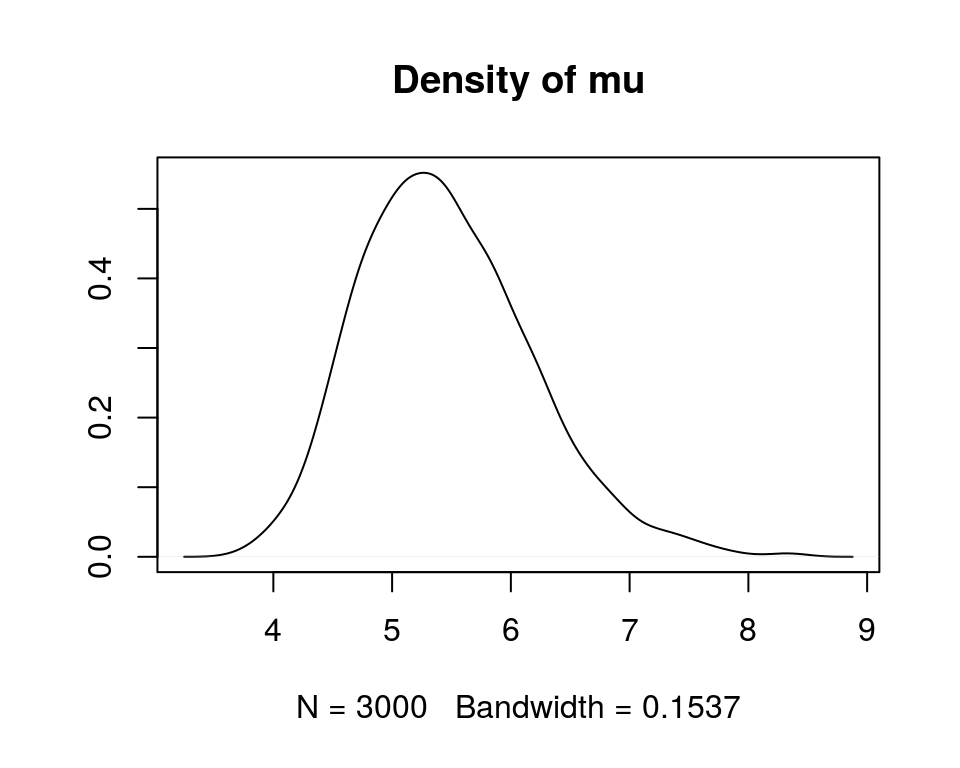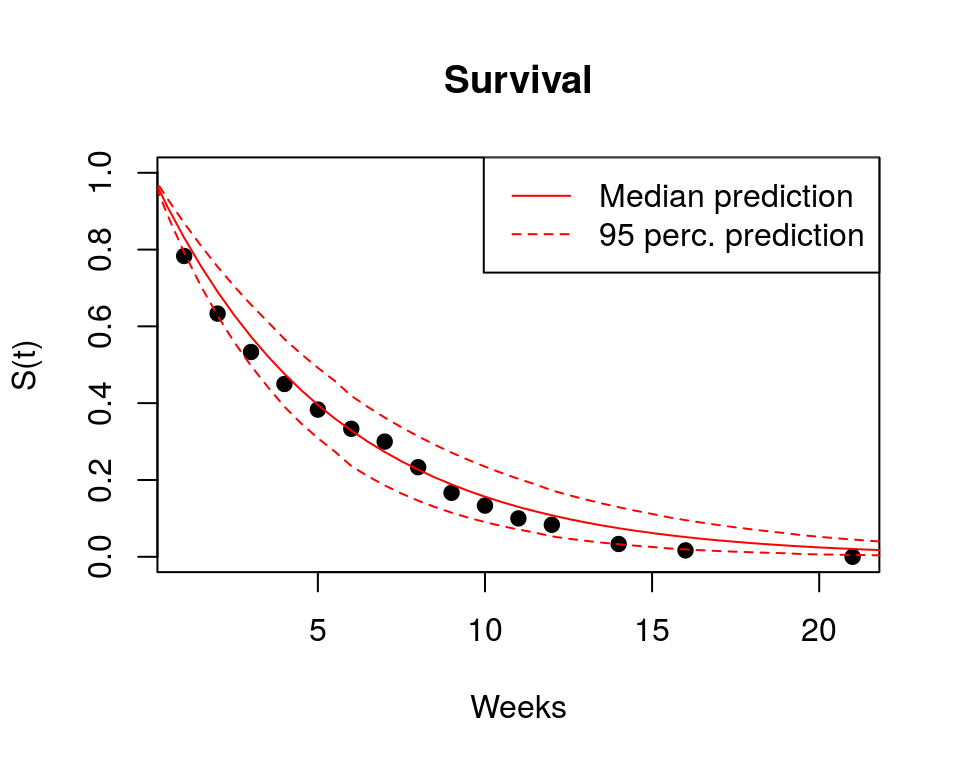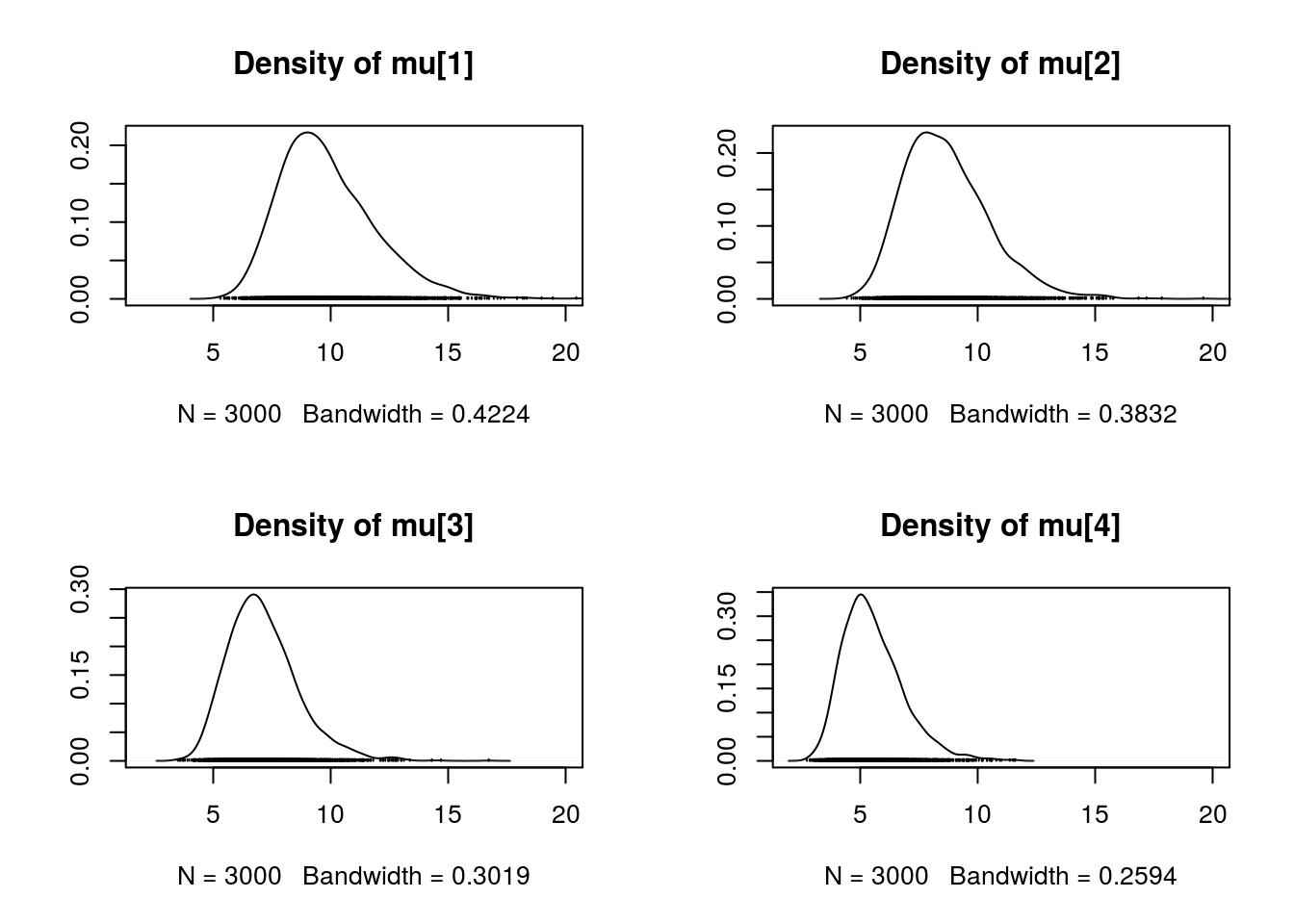# 1 Introduction and disclaimer

In this document I give elementary intro to the concepts of survival analysis, and I also provide some simple R and JAGS examples. I created this in order to learn the basics myslef – I am not an expert, so please use this critically.

The main sources that I heavily relied on are:

• Crawley (2007) The R book, chapter 25 - Survival Analysis (I use the data and many didactic formulations).

• I am grateful to the authors of this document (Joseph G. Ibrahim) and this document (G. Rodríguez) for nice expositions.

• The Wikipedia article on Survival analysis.

# 2 Concepts and definitions

Let’s assume that all failures happen continuously along the time ($$t$$) axis, so that the following reasoning will use rules for continous functions. The definitions for discrete time steps would look somewhat different.

Survival cumulative distribution function $$S(t)$$ (or survivor function, survivorship function or reliability function) gives the cumulative probability of survival of an individual along the time ($$t$$) axis:

$S(t)=Pr(T>t)$

where $$T$$ is random variable denoting time to death, and $$Pr$$ is probability that the time to death is later than some specified time $$t$$. Usually $$S(0)=1$$ and $$S(t) \to 0$$ as $$t \to \infty$$. $$S(t)$$ must be non-increasing, and so $$S(u) \leq S(t)$$ if $$u \geq t$$. Sometimes an alternative definition can be found in the literature: $$S(t)=Pr(T \geq t)$$.

Failure cumulative distribution function $$F(t)$$ is the complement of survival function, and hence it gives the cumulative probability of failure (death) along the $$t$$ axis:

$F(t)=Pr(T \leq t) = 1-S(t)$

The derivative of $$F$$ is failure probability density function $$f(t)$$, which is the rate of failures (deaths) per unit time:

$f(t)=F'(t)=\frac{d}{dt}F(t)$

Note that the relationship between $$f(t)$$ and $$F(t)$$ is the basic relation between any continuous probability density function and its cumulative distribution function!

Hazard function ($$\lambda$$) (also force of mortality or hazard rate or hazard or instantaneous failure rate or age-specific failure rate) is the event rate at time $$t$$, conditional on survival until time $$t$$ or later (that is, $$T \geq t$$); it is also the ratio of $$f(t)$$ and $$S(t)$$:

$\lambda(t)=\lim_{\Delta t \to 0} \frac{Pr(t \leq T < (t + \Delta t))}{\Delta t \cdot S(t)} = \frac{f(t)}{S(t)} =-\frac{S'(t)}{S(t)}$

$$\lambda(t)$$ must be positive, $$\lambda(t) \geq 0$$ and its integral over $$[0, \infty]$$ must be infinite. $$\lambda(t)$$ can be increasing or decreasing, or even discontinuous. In the equation above it is, however, defined for continuous $$\lambda(t)$$.

Cumulative hazard function ($$\Lambda$$) is an alternative expression of the hazard function:

$\Lambda(t)=-\log S(t) = \int_0^t \lambda(u)\,du$

where $$u \geq t$$. Transposing signs and exponentiating

$S(t)=e ^ {-\Lambda(t)}$

or differentiating (with the chain rule)

$\frac{d}{dt}\Lambda(t)=-\frac{S'(t)}{S(t)}= \lambda(t)$

Mean time to death ($$\mu$$) for continuous $$S(t)$$ is:

$\mu =\! \int_0^\infty uf(u)du$

This can also be written as $\mu =\! \int_0^\infty S(u)du$ which can be easier to deal with (the proof is here).

# 3 Exponentially declining $$f(t)$$

When $$\lambda(t)$$ is independent on age, then the probability density for the proportion of the original cohort at $$t$$ declines exponentially:

$f(t)=\frac{e^{-t/\mu}}{\mu} = \lambda e^{-\lambda t}$

where both $$\mu > 0$$ and $$t > 0$$. Note that $$f(t)$$ has an intercept at $$1/\mu$$ (because $$e^0=1$$). In other words, the number from the initial cohort dying per unit time declines exponentially with time, and a fraction $$1/\mu$$ dies during the first time interval (and, indeed, during every subsequent time interval).

Survival cumulative distribution function (i.e. the proportion of individuals from the initial cohort that are still alive at time $$t$$) is:

$S(t)=\! \int_t^\infty f(u)du=e^{-t/\mu}=e^{-\lambda t}$

$$S(t)$$ has an intercept at 1 (all the cohort is alive at time 0), and shows the probability of surviving at least as long as $$t$$.

The hazard function is then:

$\lambda(t)=\frac{f(t)}{S(t)}=\frac{e^{-t/\mu}}{\mu {e}^{-t/\mu}}=\frac{1}{\mu}= \lambda$

Which is constant hazard! Thus, for exponential $$f(t)$$ the hazard is the reciprocal of the mean time to death, and vice versa.

Finally:

$\Lambda(t)= \! \int_0^t \lambda(u)du = \! \int_0^t \lambda du = \lambda t$

The mean is then simply

$\mu = \int_0^\infty u \lambda e^{-\lambda u} du = \frac{1}{\lambda}$

For more complex $$f(t)$$ look for Weibull distribution, which generalizes the exponential.

# 5 Fitting the exponential model using package survival

## 5.1 The seedlings data

The data come from Crawley (2007) The R book; all of the datasets used in the book can be found here. Specifically, I will use the seedlings.txt example, which Crawley also uses for his demonstrations.

  seedlings <- read.table("http://goo.gl/chMvEo", header=TRUE)

The data have three colums:

cohort – month in which the seedlings were planted.

death – week at which the seedling died.

gapsize – size of canopy gap at which germination occurred.

  summary(seedlings)
##        cohort       death           gapsize
##  October  :30   Min.   : 1.000   Min.   :0.0291
##  September:30   1st Qu.: 2.000   1st Qu.:0.3982
##                 Median : 4.000   Median :0.6955
##                 Mean   : 5.367   Mean   :0.6144
##                 3rd Qu.: 8.000   3rd Qu.:0.8693
##                 Max.   :21.000   Max.   :0.9878
  attach(seedlings)

A common practice in survival analysis is to indicate if the observed times to death are censored. In the seedlings data there are no censored observations, which I will indicate by creating a status variable containing only 1s:

  status <- 1*(death>0)
status # there are no censored observations
##   1 1 1 1 1 1 1 1 1 1 1 1 1 1 1 1 1 1 1 1 1 1 1 1 1 1 1 1 1 1 1 1 1 1 1
##  1 1 1 1 1 1 1 1 1 1 1 1 1 1 1 1 1 1 1 1 1 1 1 1 1

Note the use of the status variable in the cancer example below, where we use real censored data, and where the status vector contains 0s and 1s.

## 5.2 The model fitting

survival package is the generic tool to do survival analysis in R.

  library(survival)

I will use function survreg to fit parametric survival model with the ~1 indicating that I am only fitting the intecept – there will be no predictors or groups in the model.

  model.par <- survreg(Surv(death)~1, dist="exponential")
model.par
## Call:
## survreg(formula = Surv(death) ~ 1, dist = "exponential")
##
## Coefficients:
## (Intercept)
##    1.680207
##
## Scale fixed at 1
##
## Loglik(model)= -160.8   Loglik(intercept only)= -160.8
## n= 60

To get the estimate of $$\mu$$ (mean time to death) we do a simple exponentiation:

  mu = exp(model.par$coeff) Here are some derived quantities such as survival and failure density:  time=0:25 S.t = exp(-time/mu) f.t = exp(-time/mu)/mu  ## 5.3 Plotting the data and the model First, I calculate survivorship from the raw data:  deaths <- tapply(X=status, INDEX=death, FUN = sum) survs <- (sum(deaths)-cumsum(deaths))/sum(deaths) death.data <- data.frame(day=as.numeric(names(survs)), survs=as.numeric(survs)) And here is everything plotted together. The red lines represent the fitted model, black stuff is the raw data.  par(mfrow=c(1,2)) plot(death.data, pch=19, ylab="S(t)", xlab="Weeks", main="Survival") lines(time, S.t, col="red") hist(seedlings$death, freq=FALSE, main="Failure density",
xlab="Weeks", ylim=c(0,0.15))
lines(time, f.t, col="red")# 6 Fitting the exponential model in JAGS

Here I fit exactly the same model to the same data as above, but now I use the MCMC sampler in JAGS.

Some data preparation:

  library(runjags)
library(coda)
new.t <- seq(0,25, by=0.5) # this will be used for prediction

# put the data into list for JAGS
surv.data = list(t.to.death = seedlings$death, N = nrow(seedlings), new.t = new.t, new.N = length(new.t)) Model definition in the JAGS language:  cat(" model { # prior lambda ~ dgamma(0.01, 0.01) # likelihood for(t in 1:N) { t.to.death[t] ~ dexp(lambda) } # mean time to death mu <- 1/lambda # predictions for(i in 1:new.N) { S.t[i] <- exp(-new.t[i]/mu) } } ", file="survival_exp.txt") ## 6.1 Estimating $$\mu$$ (mean time to death) I first run the model and only monitor the $$\mu$$ node:  mu <- run.jags(data = surv.data, model = "survival_exp.txt", monitor = c("mu"), sample = 1000, burnin = 1000, n.chains = 3) densplot(as.mcmc(mu), show.obs=FALSE)This is the posterior density of mean time to death $$\mu$$. Now let’s get some predictions out. ## 6.2 Predictions and 95% prediction interval of $$S(t)$$ The model is really simple and data are tiny, and so I can afford to run the MCMC again for the predictions:  S.t <- run.jags(data = surv.data, model = "survival_exp.txt", monitor = c("S.t"), sample = 2000, burnin = 1000, n.chains = 3) S.t <- summary(S.t) And here are the raw data (black dots) and the fitted model (red).  plot(death.data, pch=19, xlab="Weeks", ylab="S(t)", main="Survival", ylim=c(0,1)) lines(new.t, S.t[,'Lower95'], lty=2, col="red") lines(new.t, S.t[,'Median'], col="red") lines(new.t, S.t[,'Upper95'], lty=2, col="red") legend("topright", legend=c("Median prediction","95 perc. prediction"), lty=c(1,2), col=c("red", "red"))# 7 Censored exponential model in JAGS Censoring occurs when we don’t know the time of death (failure) for all of the individuals; this can happen, for instance, when some patients outlive an experiment, while others leave the experiment before they die (Crawley 2007). Here I use JAGS to fit a model with right-censored data. ## 7.1 The cancer data The data for this example come, again, from Crawley’s book:  cancer <- read.table("http://goo.gl/3cnoam", header=TRUE) summary(cancer) ## death treatment status ## Min. : 1.00 DrugA :30 Min. :0.0 ## 1st Qu.: 3.00 DrugB :30 1st Qu.:1.0 ## Median : 6.00 DrugC :30 Median :1.0 ## Mean : 7.55 placebo:30 Mean :0.8 ## 3rd Qu.:10.00 3rd Qu.:1.0 ## Max. :48.00 Max. :1.0 The status variable indicates if the observation is censored (0) or if it represents real time to death (1). ## 7.2 Challenges of censoring in JAGS Censoring in JAGS is done with dinterval distribution, and it takes some time to get the idea how it works. I recommend to study the censoring section in JAGS user manual, as well as this Martyn Plummer’s presentation, slide 14. Some hard-earned insights: • Following the OpenBugs’ Mice example, individuals who are censored should be given a missing value in the vector of failure times t.to.death (see the code below), whilst individuals who fail are given a zero in the censoring time vector t.cen. • Also, citing this post, censored data must be recorded as NA, not as the value of censoring limit! • When explicitly initializing the chains, the censored values of the data must be explicitly initialized (to values above the censoring limits)! However, this was not an issue for me. Here is how I prepare the data for JAGS, having in mind the points above:  censored <- cancer$status==0
is.censored <- censored*1
t.to.death <- cancer$death t.to.death[censored] <- NA t.to.death ##  4 26 2 25 7 NA 5 NA 4 1 10 48 4 3 17 2 NA 13 7 NA 8 5 2 ##  2 4 NA 22 1 9 6 NA 8 11 NA 1 6 4 4 7 12 16 NA 19 NA 19 10 ##  3 10 11 16 1 6 1 12 1 4 NA 2 14 11 1 4 16 3 12 NA 1 16 5 ##  8 1 7 NA NA 12 19 3 NA 8 4 15 4 4 5 4 2 NA 9 3 4 1 NA ##  1 8 NA 4 NA 1 NA 9 4 NA 3 9 5 4 4 NA 13 4 NA 2 2 9 NA ##  9 NA 4 5 9  t.cen <- rep(0, times=length(censored)) t.cen[censored] <- cancer$death[censored]
t.cen
##     0  0  0  0  0  6  0  2  0  0  0  0  0  0  0  0  8  0  0 10  0  0  0
##    0  0 26  0  0  0  0 10  0  0 11  0  0  0  0  0  0  0  8  0  3  0  0
##    0  0  0  0  0  0  0  0  0  0 14  0  0  0  0  0  0  0  0  4  0  0  0
##    0  0  0  2  1  0  0  0  4  0  0  0  0  0  0  0  0  7  0  0  0  0  6
##    0  0  3  0 10  0  7  0  0 12  0  0  0  0  0  7  0  0  7  0  0  0  6
##   0  6  0  0  0
  # put the data together for JAGS
cancer.data <- list(t.to.death = t.to.death,
t.cen = t.cen,
N = nrow(cancer),
group = rep(1:4, each=30))

## 7.3 The model

The model assumes that survival in each of the four groups (indexed by $$i$$) is modelled by a stand-alone exponential model with its own $$\lambda_i$$.

Here is the model definition in JAGS, with the censoring modelled by the dinterval function:

  cat("
model
{
# priors
for(j in 1:4)
{
# prior lambda for each group
lambda[j] ~ dgamma(0.001, 0.001)
mu[j] <- 1/lambda[j] # mean time to death
}
# likelihood
for(i in 1:N)
{
is.censored[i] ~ dinterval(t.to.death[i], t.cen[i])
t.to.death[i] ~ dexp(lambda[group[i]])
}
}
", file="survival_cancer.txt")

Running the model and monitoring the $$\mu$$ node:

  library(runjags)
library(coda)

cancer.fit <- run.jags(data = cancer.data,
model = "survival_cancer.txt",
monitor = c("mu"),
sample = 1000, burnin = 1000, n.chains = 3)

And here are the posterior densities for each group’s mean time to death $$\mu$$:

  par(mfrow=c(2,2))
densplot(as.mcmc(cancer.fit), xlim=c(2,20))mu[1:3] are for the treatments, mu is the placebo.